# RD Sharma Solutions for Class 8 Maths Chapter 17 Understanding Shapes- III (Special Types of Quadrilaterals) Exercise 17.2

This exercise mainly deals with the properties of a rhombus. By referring to solutions when solving the problems from the RD Sharma textbook, students gain overall knowledge covered in this exercise. From the exam point of view, solutions are prepared using shortcut methods which speed up the ability to solve problems. Students may keep the textbook aside and use RD Sharma Solutions for revision purposes. Students can refer and download the Pdf from the links provided below.

## Download the Pdf of RD Sharma Solutions for Class 8 Maths Exercise 17.2 Chapter 17 Understanding Shapes- III (Special Types of Quadrilaterals)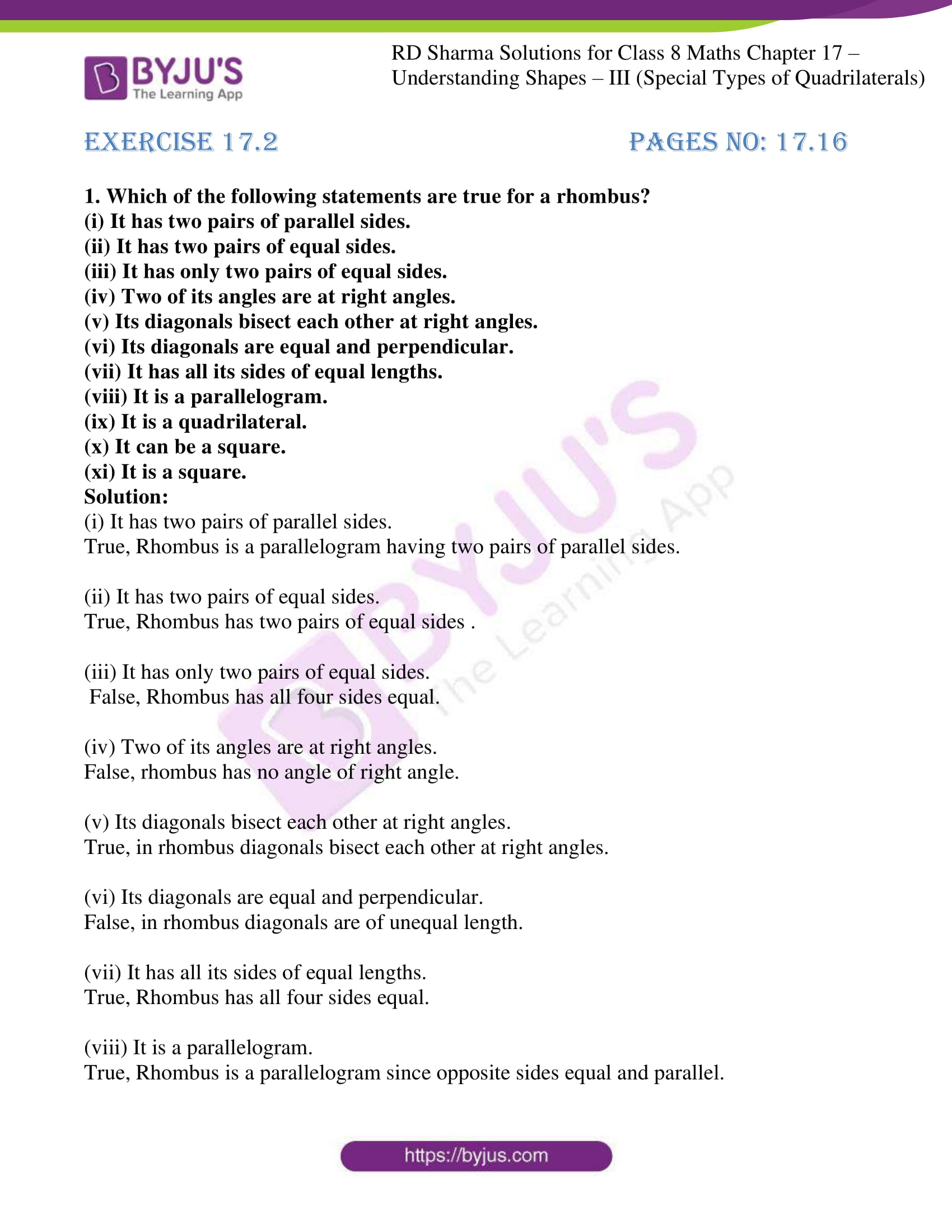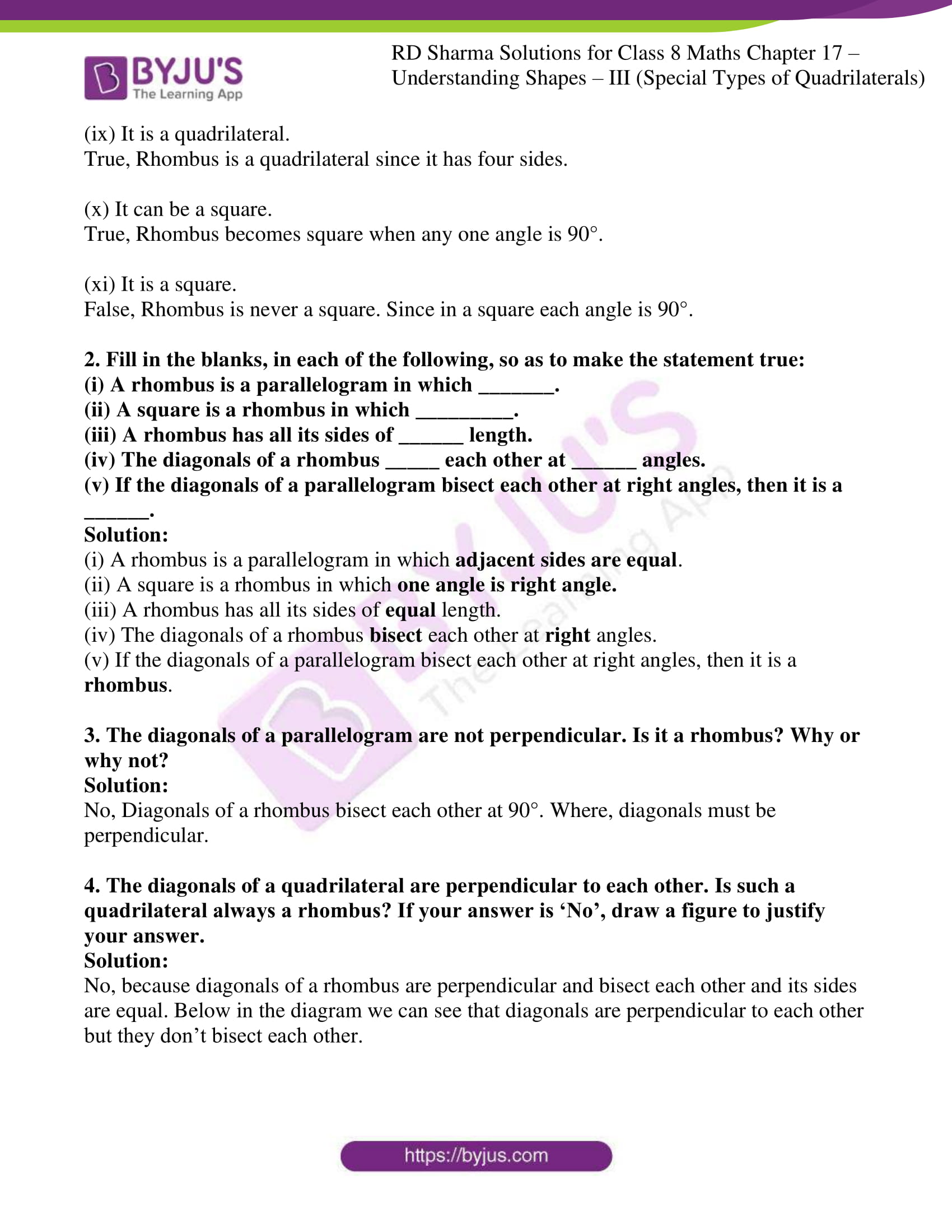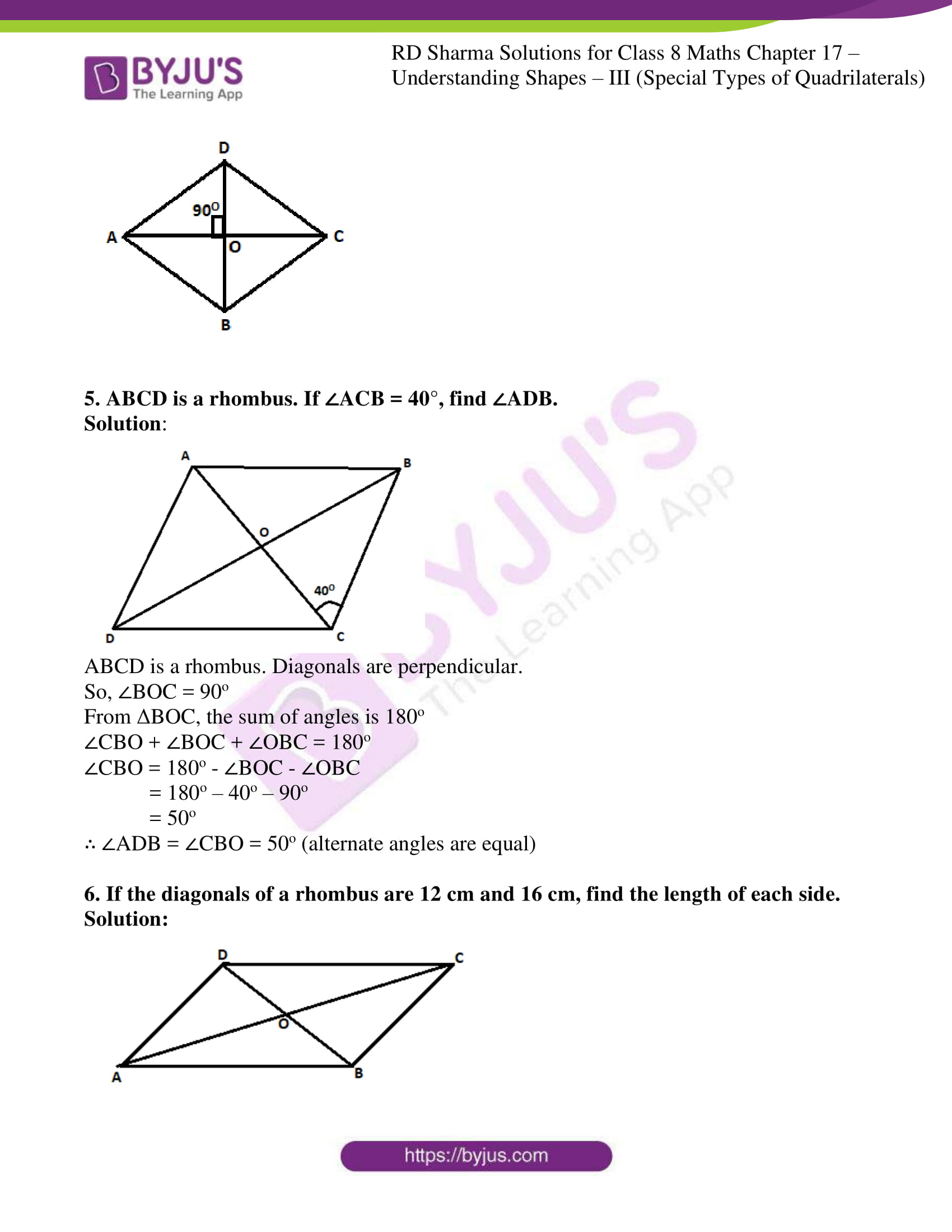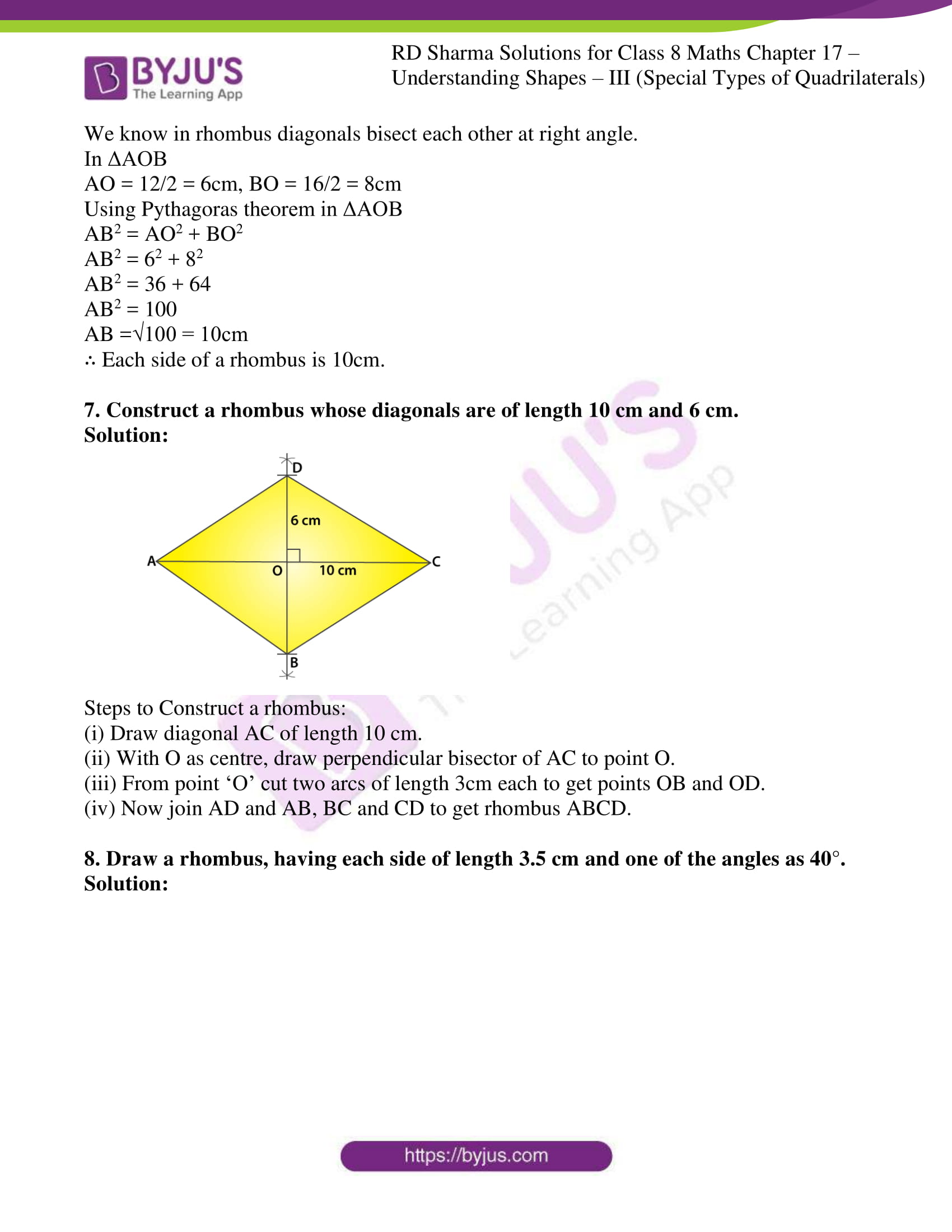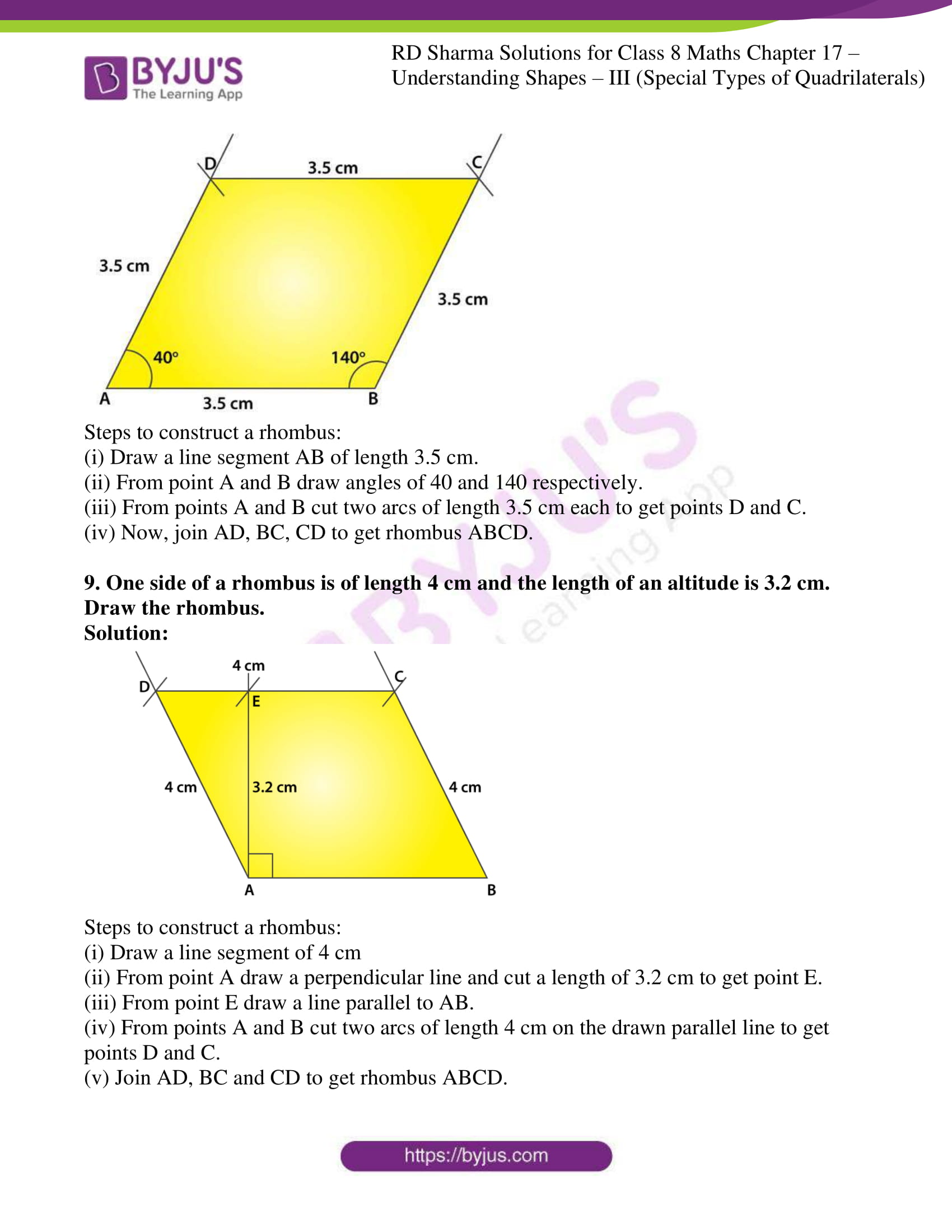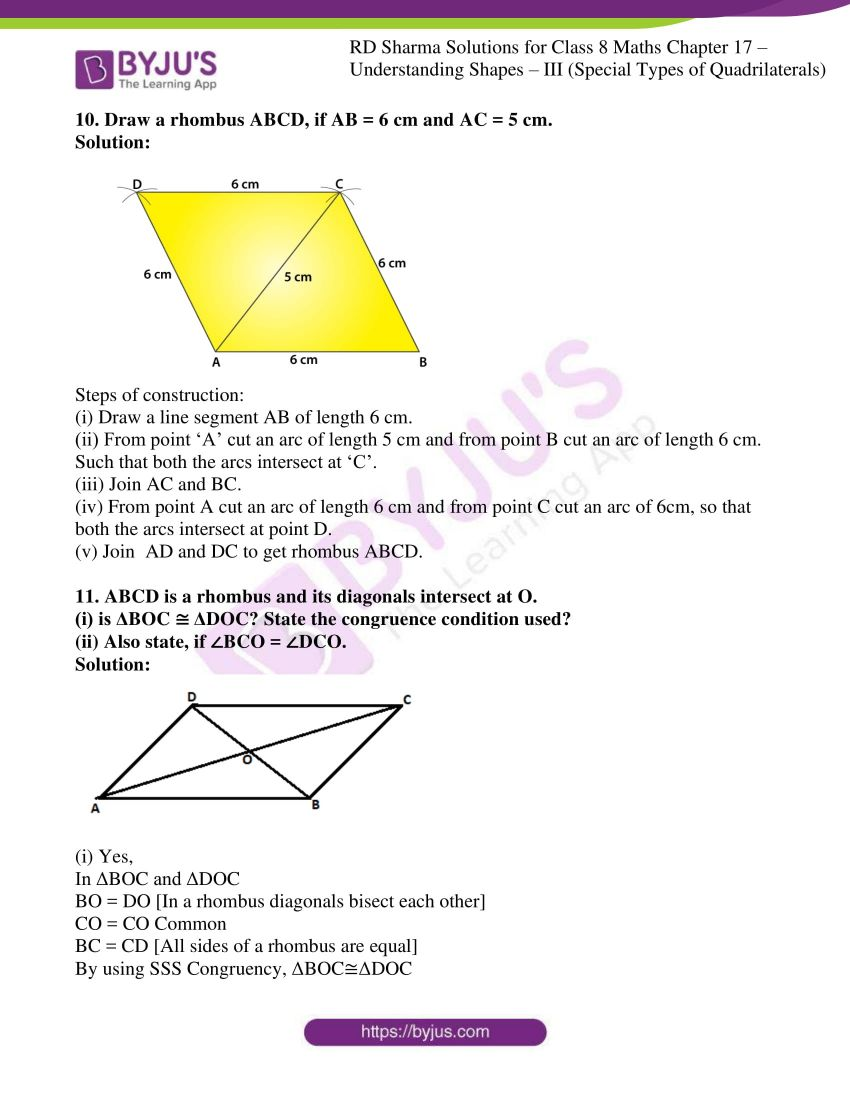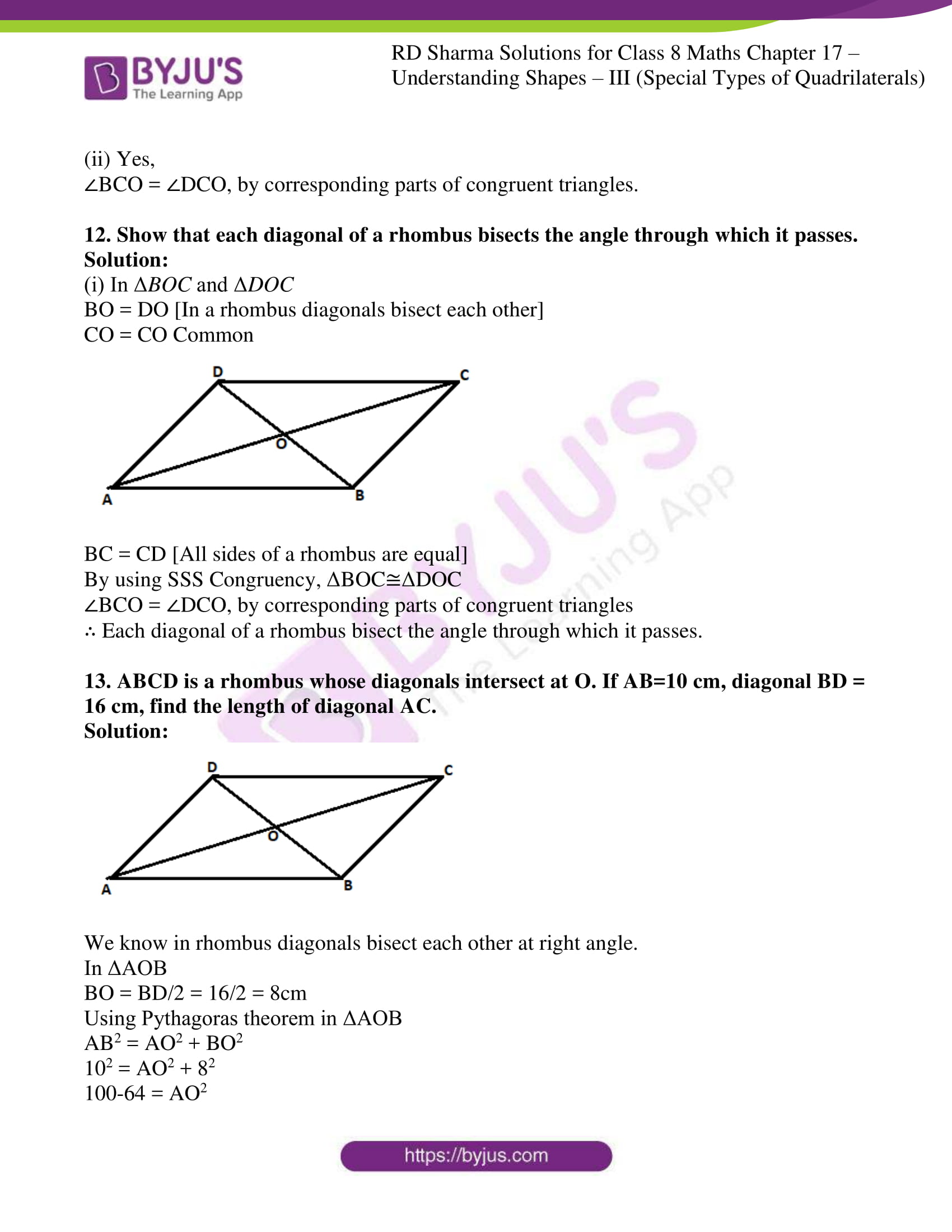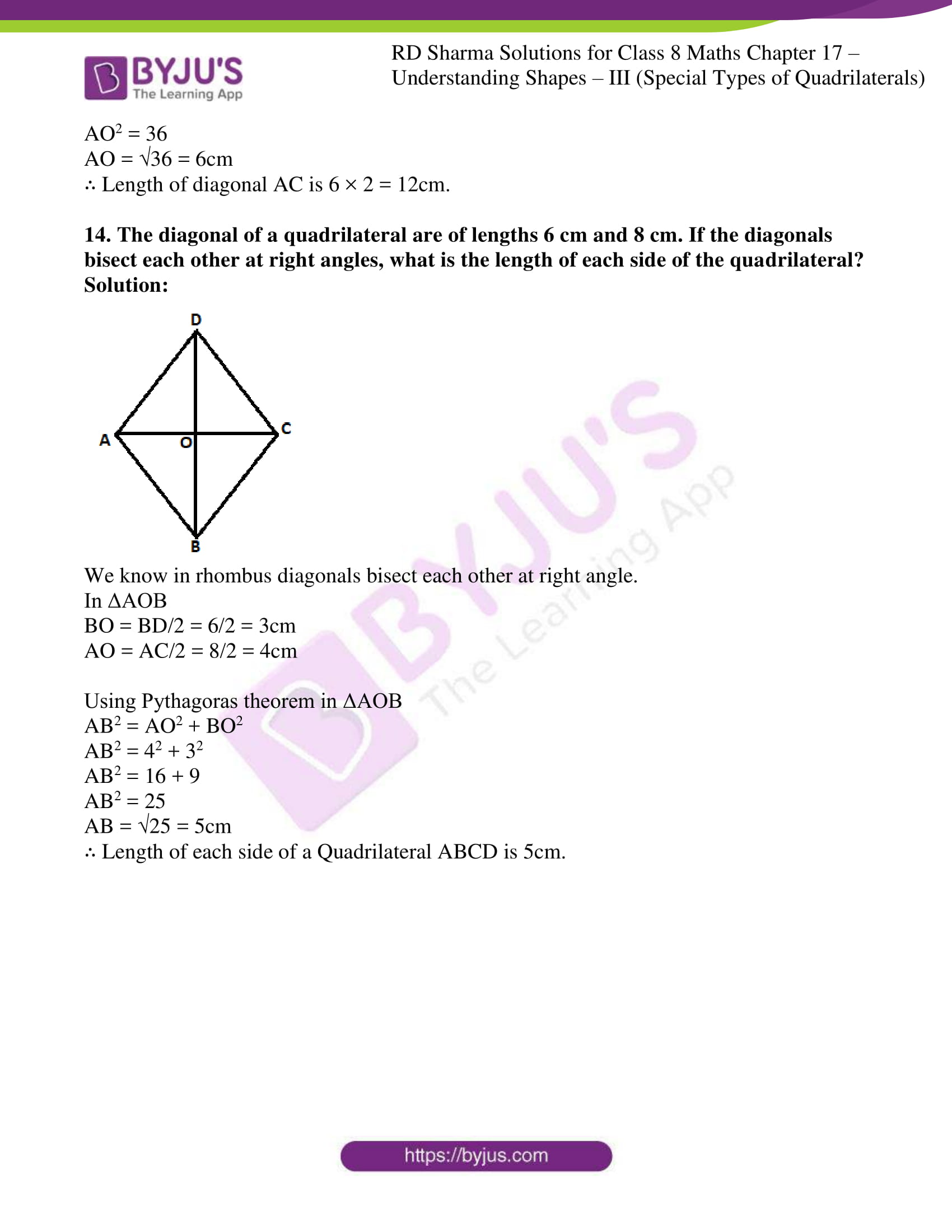### Access answers to Maths RD Sharma Solutions For Class 8 Exercise 17.2 Chapter 17 Understanding Shapes- III (Special Types of Quadrilaterals)

1. Which of the following stat
ements are true for a rhombus?
(i) It has two pairs of parallel sides.
(ii) It has two pairs of equal sides.
(iii) It has only two pairs of equal sides.
(iv) Two of its angles are at right angles.
(v) Its diagonals bisect each other at right angles.
(vi) Its diagonals are equal and perpendicular.
(vii) It has all its sides of equal lengths.
(viii) It is a parallelogram.
(x) It can be a square.
(xi) It is a square.

Solution:

(i) It has two pairs of parallel sides.

True, Rhombus is a parallelogram having two pairs of parallel sides.

(ii) It has two pairs of equal sides.

True, Rhombus has two pairs of equal sides .

(iii) It has only two pairs of equal sides.

False, Rhombus has all four sides equal.

(iv) Two of its angles are at right angles.

False, rhombus has no angle of right angle.

(v) Its diagonals bisect each other at right angles.

True, in rhombus diagonals bisect each other at right angles.

(vi) Its diagonals are equal and perpendicular.

False, in rhombus diagonals are of unequal length.

(vii) It has all its sides of equal lengths.

True, Rhombus has all four sides equal.

(viii) It is a parallelogram.

True, Rhombus is a parallelogram since opposite sides equal and parallel.

True, Rhombus is a quadrilateral since it has four sides.

(x) It can be a square.

True, Rhombus becomes square when any one angle is 90°.

(xi) It is a square.

False, Rhombus is never a square. Since in a square each angle is 90°.

2. Fill in the blanks, in each of the following, so as to make the statement true:
(i) A rhombus is a parallelogram in which _______.
(ii) A square is a rhombus in which _________.
(iii) A rhombus has all its sides of ______ length.
(iv) The diagonals of a rhombus _____ each other at ______ angles.
(v) If the diagonals of a parallelogram bisect each other at right angles, then it is a ______.

Solution:

(i) A rhombus is a parallelogram in which adjacent sides are equal.

(ii) A square is a rhombus in which one angle is right angle.

(iii) A rhombus has all its sides of equal length.

(iv) The diagonals of a rhombus bisect each other at right angles.

(v) If the diagonals of a parallelogram bisect each other at right angles, then it is a rhombus.

3. The diagonals of a parallelogram are not perpendicular. Is it a rhombus? Why or why not?

Solution:

No, Diagonals of a rhombus bisect each other at 90°. Where, diagonals must be perpendicular.

Solution:

No, because diagonals of a rhombus are perpendicular and bisect each other and its sides are equal. Below in the diagram we can see that diagonals are perpendicular to each other but they don’t bisect each other.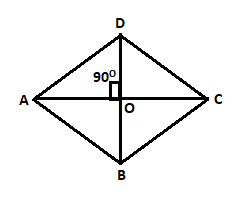5. ABCD is a rhombus. If ∠ACB = 40°, find ∠ADB.

Solution: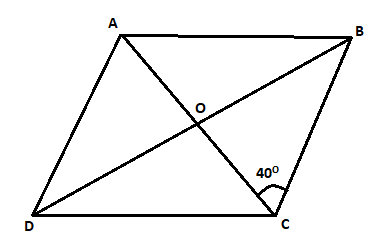ABCD is a rhombus. Diagonals are perpendicular.

So, ∠BOC = 90o

From ΔBOC, the sum of angles is 180o

∠CBO + ∠BOC + ∠OBC = 180o

∠CBO = 180o – ∠BOC – ∠OBC

= 180o – 40o – 90o

= 50o

∴ ∠ADB = ∠CBO = 50o (alternate angles are equal)

6. If the diagonals of a rhombus are 12 cm and 16 cm, find the length of each side.

Solution: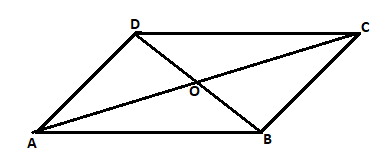We know in rhombus diagonals bisect each other at right angle.

In ΔAOB

AO = 12/2 = 6cm, BO = 16/2 = 8cm

Using Pythagoras theorem in ΔAOB

AB2 = AO2 + BO2

AB2 = 62 + 82

AB2 = 36 + 64

AB2 = 100

AB =√100 = 10cm

∴ Each side of a rhombus is 10cm.

7. Construct a rhombus whose diagonals are of length 10 cm and 6 cm.

Solution: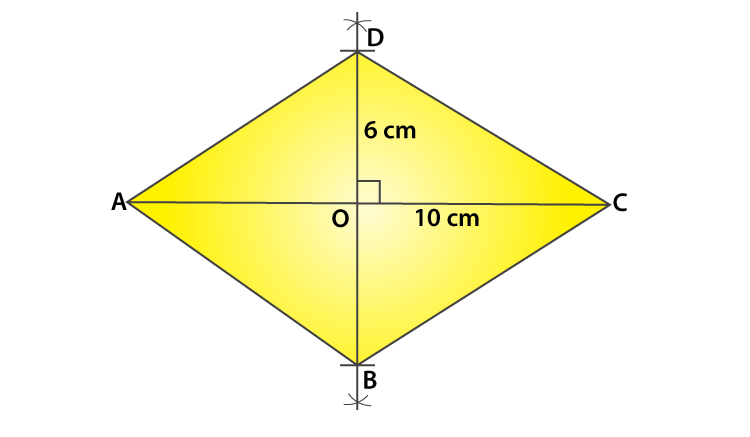Steps to Construct a rhombus:

(i) Draw diagonal AC of length 10 cm.

(ii) With O as centre, draw perpendicular bisector of AC to point O.

(iii) From point ‘O’ cut two arcs of length 3cm each to get points OB and OD.

(iv) Now join AD and AB, BC and CD to get rhombus ABCD.

8. Draw a rhombus, having each side of length 3.5 cm and one of the angles as 40°.

Solution: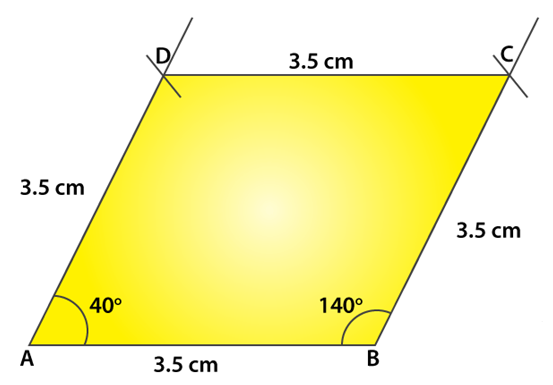Steps to construct a rhombus:

(i) Draw a line segment AB of length 3.5 cm.

(ii) From point A and B draw angles of 40 and 140 respectively.

(iii) From points A and B cut two arcs of length 3.5 cm each to get points D and C.

(iv) Now, join AD, BC, CD to get rhombus ABCD.

9. One side of a rhombus is of length 4 cm and the length of an altitude is 3.2 cm. Draw the rhombus.

Solution: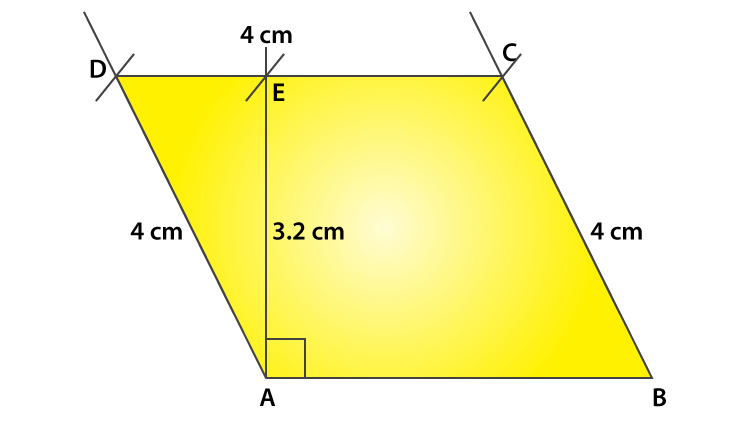Steps to construct a rhombus:

(i) Draw a line segment of 4 cm

(ii) From point A draw a perpendicular line and cut a length of 3.2 cm to get point E.

(iii) From point E draw a line parallel to AB.

(iv) From points A and B cut two arcs of length 4 cm on the drawn parallel line to get points D and C.

(v) Join AD, BC and CD to get rhombus ABCD.

10. Draw a rhombus ABCD, if AB = 6 cm and AC = 5 cm.

Solution: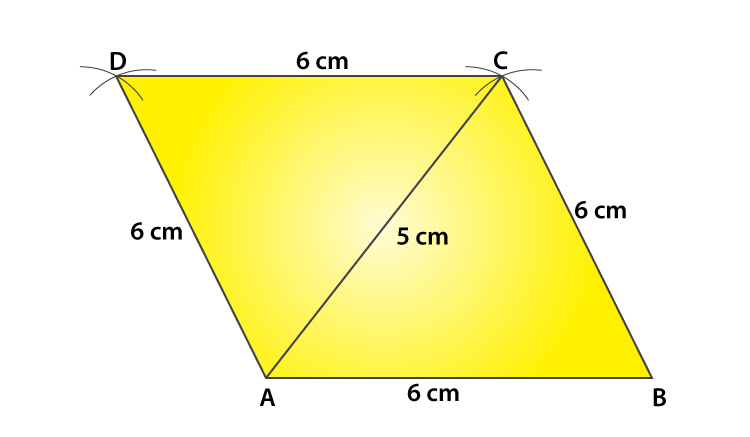Steps of construction:

(i) Draw a line segment AB of length 6 cm.

(ii) From point ‘A’ cut an arc of length 5 cm and from point B cut an arc of length 6 cm. Such that both the arcs intersect at ‘C’.

(iii) Join AC and BC.

(iv) From point A cut an arc of length 6 cm and from point C cut an arc of 6cm, so that both the arcs intersect at point D.

(v) Join AD and DC to get rhombus ABCD.

11. ABCD is a rhombus and its diagonals intersect at O.
(i) is ΔBOC ≅ ΔDOC? State the congruence condition used?
(ii) Also state, if ∠BCO = ∠DCO.

Solution:(i) Yes,

In ΔBOC and ΔDOC

BO = DO [In a rhombus diagonals bisect each other]

CO = CO Common

BC = CD [All sides of a rhombus are equal]

By using SSS Congruency, ΔBOC≅ΔDOC

(ii) Yes,

∠BCO = ∠DCO, by corresponding parts of congruent triangles.

12. Show that each diagonal of a rhombus bisects the angle through which it passes.

Solution:

(i) In ΔBOC and ΔDOC

BO = DO [In a rhombus diagonals bisect each other]

CO = CO Common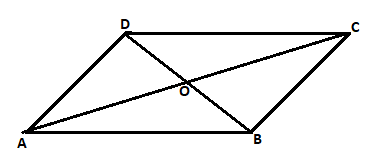BC = CD [All sides of a rhombus are equal]

By using SSS Congruency, ΔBOC≅ΔDOC

∠BCO = ∠DCO, by corresponding parts of congruent triangles

∴ Each diagonal of a rhombus bisect the angle through which it passes.

13. ABCD is a rhombus whose diagonals intersect at O. If AB=10 cm, diagonal BD = 16 cm, find the length of diagonal AC.

Solution:We know in rhombus diagonals bisect each other at right angle.

In ΔAOB

BO = BD/2 = 16/2 = 8cm

Using Pythagoras theorem in ΔAOB

AB2 = AO2 + BO2

102 = AO2 + 82

100-64 = AO2

AO2 = 36

AO = √36 = 6cm

∴ Length of diagonal AC is 6 × 2 = 12cm.

14. The diagonal of a quadrilateral are of lengths 6 cm and 8 cm. If the diagonals bisect each other at right angles, what is the length of each side of the quadrilateral?

Solution: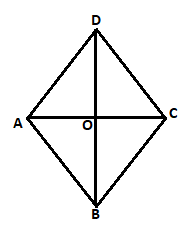We know in rhombus diagonals bisect each other at right angle.

In ΔAOB

BO = BD/2 = 6/2 = 3cm

AO = AC/2 = 8/2 = 4cm

Using Pythagoras theorem in ΔAOB

AB2 = AO2 + BO2

AB2 = 42 + 32

AB2 = 16 + 9

AB2 = 25

AB = √25 = 5cm

∴ Length of each side of a Quadrilateral ABCD is 5cm.